sea_ljf

## Self-balanced Binary Search Trees with AVL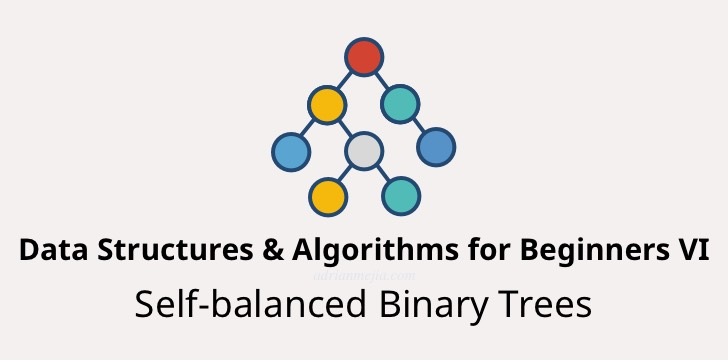Binary Search Trees are used for many things that we might not be aware of. For instance: Website’s databases use trees to search data more efficiently. HTML DOM elements are represented as a tree. For trees to be effective they need to be balanced. So, we are going to discuss how to keep the BST balanced as you add and remove elements.

In this post, we are going to explore different techniques to balance a tree. We are going to use rotations to move nodes around and the AVL algorithm to keep track if the tree is balanced or needs adjustments. Let’s dig in!

This post is part of a tutorial series:

Learning Data Structures and Algorithms (DSA) for Beginners

Let’s start by defining what is a balanced tree and the pitfalls of an unbalanced tree.

# Balanced vs Unbalanced Binary Search Tree

As discussed in the previous post the worst nightmare for a BST is to be given numbers in order (e.g. 1, 2, 3, 4, 5, 6, 7, …).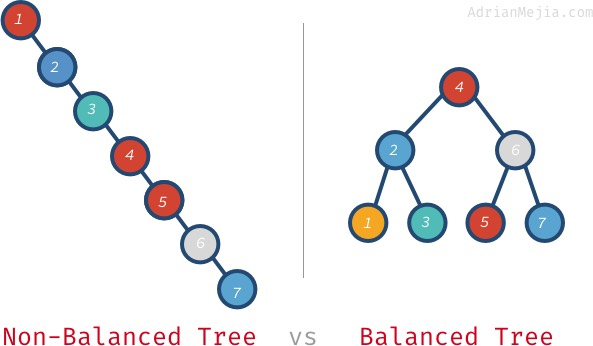If we ended up with a tree like the one on the left we are screwed. This is because to find out if a node is on the tree or not you will have to visit every node. That takes O(n), while if we keep the node balanced in every insertion or deletion we could have O(log n).

Again, this might not look like a big difference but when you have a million nodes the difference is abysmal. We are talking about visiting 1,000,000 nodes vs visiting 20!

“Ok, I’m sold. How do I keep the tree balanced?” you might ask. Well, let’s first learn when to tell that a tree is unbalanced.

# When a tree is balanced/non-balanced?

Take a look at the following trees and tell which one is balanced and which one is not.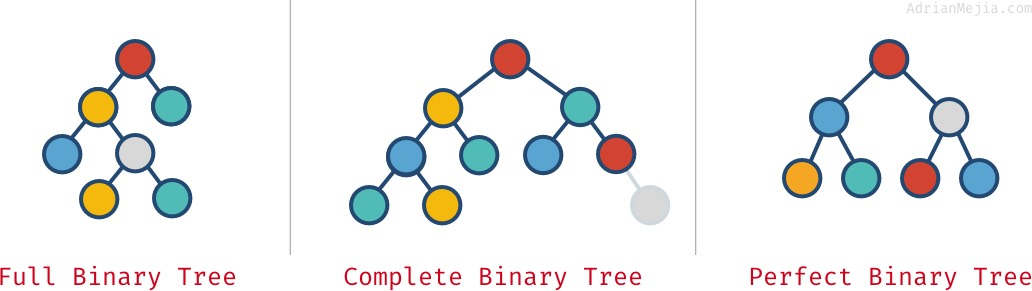Well, a tree is definately balanced when is a perfect tree (all the levels on the tree have maximum number of nodes). But what about full trees or complete trees ?

The “complete tree” looks somewhat balanced, right? What about the full tree? Well, it starts to get tricky. Let’s work on a definition.

A tree is balanced if:

1. The left subtree height and the right subtree height differ by at most 1.
2. Visit every node making sure rule #1 is satisfied.

For instance, if you have a tree with 7 nodes:

|

``````1234567
``````

|

``````    10   /   \  5    20 /     / \4    15   30     /    12
``````

|

If you check the subtrees’ heights (edge counts to farthest leave) recursively you will notice they never differ by more than one.

• 10 descendants:
• Left subtree 5 has a height of 1, while right subtree 20 has a height of 2. The difference is one so: Balanced!
• 20 descendants:
• Left subtree15 has a height of 1, while right subtree 30 has a height of 0. So the diff is 1: Balanced!

On the other hand, take a look at this tree:

|

``````1234567
``````

|

``````     40   /   \  35    60* /     /25    50     /    45
``````

|

Let’s check the subtrees height recursively:

• 40 descendants:
• Left subtree 35 has a height of 1, while right subtree 60 has a height of 2. The difference is one so: Balanced!
• 60 descendants:
• Left subtree 50 has a height of 2, while the right subtree (none) has a height of 0. The difference between 2 and 0 is more than one, so: NOT balanced!

Hopefully, now you can calculate balanced and unbalanced trees. What can we do when we find an unbalanced tree? We do rotations!

If we take the same tree as before and move 50 to the place of 60 we get the following:

|

``````12345
``````

|

``````     40   /   \  35    50 /     /   \25    45    60*
``````

|

After rotating 60 to the right, It’s balanced! Let’s learn all about it in the next section.

# Tree rotations

Before throwing any line of code, let’s spend some time thinking about how to balance small trees using rotations.

## Left Rotation

Let’s say that we have the following tree with ascending values: 1-2-3

|

``````12345
``````

|

``````1*                                        2 \                                       /  \  2     ---| left-rotation(1) |-->      1*   3   \    3
``````

|

To perform a left rotation on node 1, we move it down as it’s children’s (2) left descendant.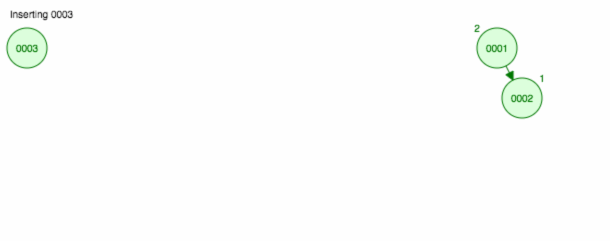This is called single left rotation or Left-Left (LL) rotation.

For the coding part, let’s do another example:

|

``````1234567
``````

|

``````1                                 1 \                                 \  2*                                3   \    --left-rotation(2)->       / \    3                             2*  4     \      4
``````

|

To define the tree we are using TreeNode that we developed in the previous post.

|

``````1234567891011
``````

|

``````const n1 = new TreeNode(1);const n2 = new TreeNode(2);const n3 = new TreeNode(3);const n4 = new TreeNode(4);n1.right = n2;n2.right = n3;n3.right = n4;const newParent = leftRotation(n2);console.log(newParent === n3);
// true
``````

|

In this case, we are rotating 2 to the left. Let’s implement the leftRotation function.

leftRotationCode

|

``````12345678910111213
``````

|

``````function leftRotation(node) {  const newParent = node.right;
// e.g. 3  const grandparent = node.parent;
// e.g. 1  // make 1 the parent of 3 (previously was the parent of 2)  swapParentChild(node, newParent, grandparent);
// do LL rotation  newParent.left = node;
// makes 2 the left child of 3  node.right = undefined;
// clean 2's right child  return newParent;
// 3 is the new parent (previously was 2)}
``````

|

Notice that we are using a utility function to swap parents called swapParentChild.

swapParentChildCode

|

``````12345678910
``````

|

``````function swapParentChild(oldChild, newChild, parent) {  if (parent) {    const side = oldChild.isParentRightChild ? 'right' : 'left';
// this set parent child AND also    parent[side] = newChild;
} else {    // no parent? so set it to null    newChild.parent = null;
}}
``````

|

We are using this function to make 1 the parent of 3. We are going to use it rotation right as well.

## Right Rotation

We have the following tree with descending values 4-3-2-1:

|

``````1234567
``````

|

``````      4                                        4     /                                        /    3*                                       2   /                                        /  \  2       ---| right-rotation(3) |-->      1    3* /1
``````

|

To perform a right rotation on node 3 we move it down as its child 2‘s right descendatnt.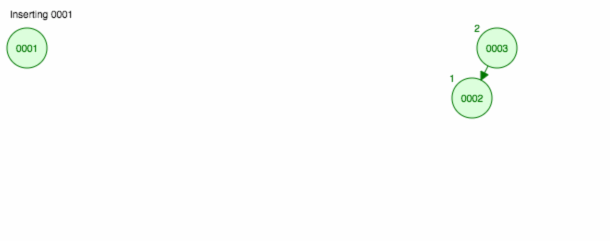This is called single right rotation or Right-Right (RR) rotation.

The code is pretty similar to what we did on the left rotation:

rightRotationCode

|

``````123456789101112
``````

|

``````function rightRotation(node) {  const newParent = node.left;
const grandparent = node.parent;
swapParentChild(node, newParent, grandparent);
// do RR rotation  newParent.right = node;
node.left = undefined;
return newParent;}
``````

|

The rightRotation does the following:

1. First, we swap 4‘s child: before it was 3 and after the swap is 2 (line 5).
2. Later, we make 3 the right child of 2 (line 8) and
3. Finally, we clean up the 3 right child reference to null (line 9).

Now that know how single rotations work to the left and right we can combine them: left-right and right-left rotations.

## Left-Right Rotation

If we insert values on a BST in this order: 3-1-2. We will get an unbalanced tree. In order to balance the tree we have to do a leftRightRotation(3).

|

``````12345
``````

|

``````  3*                                       2* /                                        /  \1    --| left-right-rotation(3) |->      1    3 \  2
``````

|

Double rotations are a combination of the other two rotations we discussed in (LL and RR):

If we expand the left-right-rotation into the two single rotations we would have:

|

``````12345
``````

|

``````  3*                          3* /                          /                            21   -left-rotation(1)->    2    -right-rotation(3)->    /  \ \                        /                            1    3*  2                      1
``````

|

• left-rotation(1): We do a left rotation on the nodes’ left child. E.g. 1
• right-rotation(3): right rotation on the same node. E.g. 3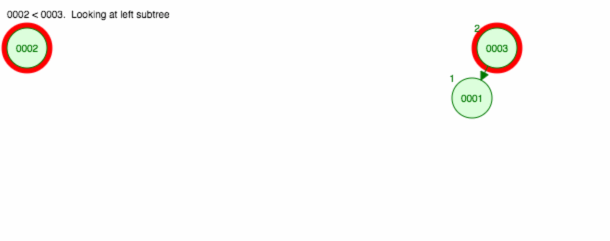This is double rotation called Left-Right (LR) rotation.

leftRightRotationCode

|

``````1234
``````

|

``````function leftRightRotation(node) {  leftRotation(node.left);
return rightRotation(node);}
``````

|

The code is very simple since we leverage the leftRotation and rightRotation that we did before.

## Right-Left Rotation

When we insert nodes on the following order: 1-3-2, we need to perform a rightLeftRotation(1) to balance the tree.

|

``````12345
``````

|

``````1*                           1* \                            \                              2   3   -right-rotation(3)->    2   -left-rotation(1)->      /  \ /                              \                          1*   32                                3
``````

|

The code to is very similar to LR rotation:

leftRightRotationCode

|

``````1234
``````

|

``````function rightLeftRotation(node) {  rightRotation(node.right);
return leftRotation(node);}
``````

|

We know all the rotations needed to balanced any binary tree. Let’s go ahead an use the AVL algorithm to keep it balanced on insertions/deletions.

# AVL Tree Overview

AVL Tree was the first self-balanced tree invented. It is named after the two inventors Adelson-Velsky and Landis. In their self-balancing algorithm if one subtree differs from the other by at most one then rebalancing is done using rotations.

We already know how to do rotations from the previous sections, the next step is to figure out the subtree’s heights. We are going to call balance factor, the diff between the left and right subtree on a given node.

balanceFactor = leftSubtreeHeight - rightSubtreeHeight

If the balance factor is bigger than 1 or less than -1 then, we know we need to balance that node. We can write the balance function as follows:

BalanceCode

|

``````1234567891011121314151617
``````

|

``````function balance(node) {  if (node.balanceFactor > 1) {    // left subtree is higher than right subtree    if (node.left.balanceFactor > 0) {      rightRotation(node);
} else if (node.left.balanceFactor < 0) {      leftRightRotation(node);
}  } else if (node.balanceFactor < -1) {    // right subtree is higher than left subtree    if (node.right.balanceFactor < 0) {      leftRotation(node);
} else if (node.right.balanceFactor > 0) {      rightLeftRotation(node);
}  }}
``````

|

Based on the balance factor there 4 different rotation that we can do: RR, LL, RL, and LR. To know what rotation to do we:

1. Take a look into the given node‘s balanceFactor.
2. If balance factor is -1, 0 or 1 we are done.
3. If the node needs balancing, then we use the node’s left or right balance factor to tell which kind of rotation it needs.

Notice that we haven’t implemented the node.balanceFactor attribute yet, but we are going to do that next.

One of the easiest ways to implement subtree heights is using recursion. Let’s go ahead and add height-related properties to TreeNode class:

height, leftSubtreeHeight and rightSubtreeHeightCode

|

``````123456789101112131415
``````

|

``````get height() {  return Math.max(this.leftSubtreeHeight, this.rightSubtreeHeight);}get leftSubtreeHeight() {  return this.left ? this.left.height + 1 : 0;}get rightSubtreeHeight() {  return this.right ? this.right.height + 1 : 0;}get balanceFactor() {  return this.leftSubtreeHeight - this.rightSubtreeHeight;}
``````

|

To understand better what’s going on let’s do some examples.

## Tree with 1 node

|

``````12
``````

|

``````  40*/     \
``````

|

• Since this node doesn’t have left nor right children then leftSubtreeHeight and rightSubtreeHeight will return 0.
• Height is Math.max(this.leftSubtreeHeight, this.rightSubtreeHeight) which is Math.max(0, 0), so height is 0.
• Balance factor is also zero since 0 - 0 = 0.

## Tree with multiple nodes

Let’s try with multiple nodes

|

``````1234567
``````

|

``````     40   /   \  35    60 /     /25    50     /    45
``````

|

balanceFactor(45)

• As we saw leaf nodes doesn’t have left or right subtree so their heights are 0, thus balance factor is 0.

balanceFactor(50)

• leftSubtreeHeight = 1 and rightSubtreeHeight = 0.
• height = Math.max(1, 0), so it’s 1.
• Balance factor is 1 - 0, so it’s 1 as well.

balanceFactor(60)

• leftSubtreeHeight = 2 and rightSubtreeHeight = 0.
• height = Math.max(2, 0), so it’s 2.
• Balance factor is 2 - 0, so it’s 2 and it’s UNBALANCED!

If we use our balance function on node 60 that we developed, then it would do a rightRotation on 60 and the tree will look like:

|

``````12345
``````

|

``````     40   /   \  35    50 /     /   \25    45    60*
``````

|

Before the height of the tree (from the root) was 3, now it’s only 2.

Let’s put all together and explain how we can keep a binary search tree balanced on insertion and deletion.

# AVL Tree Insertion and Deletion

AVL tree is just a layer on top of a regular Binary Search Tree (BST). The add/remove operations are the same as in the BST, the only difference is that we run the balance function after each operation.

Let’s implement the AVL Tree.

AvlTreeCode

|

``````123456789101112131415161718192021
``````

|

``````const BinarySearchTree = require('./binary-search-tree');const { balanceUptream } = require('./tree-rotations');class AvlTree extends BinarySearchTree {  add(value) {    const node = super.add(value);
balanceUptream(node);
return node;
}  remove(value) {    const node = super.find(value);
if (node) {      const found = super.remove(value);
balanceUptream(node.parent);
return found;
}    return false;
}}
``````

|

If you need to review the dependencies here are the links to the implementations:

The balanceUpstream function gets executed after an insertion or deletion.

balanceUptreamContext

|

``````1234567
``````

|

``````function balanceUptream(node) {  let current = node;
while (current) {    balance(current);
current = current.parent;
}}
``````

|

We go recursively using the balance function on the nodes’ parent until we reach the root node.

In the following animation we can see AVL tree insertions and deletions in action:You can also check the test files to see more detailed examples of how to use the AVL trees.

That’s all folks!

# Summary

In this post, we explored the AVL tree which is an a special binary search tree that self-balance itself after insertions and deletions of nodes. The operations of balancing a tree involves rotations and they can be single or double rotations.

Single rotations:

• Left rotation
• Right rotation

Double rotations:

• Left-Right rotation
• Right-Left rotation

You can find all the code developed here in the Github. You can star it to keep it handy.